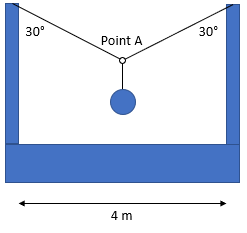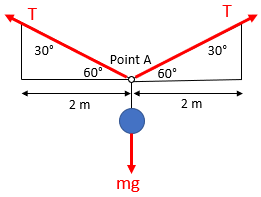## Wrecking Ball Hoist

In the below diagram, two cables merge together at point A to hoist up a 200 kg wrecking ball. What is the tension in the right cable?Hint
Free Body Diagram:Hint 2
First, evaluate the sum of forces about Point A. Keep in mind that $$Force=mass\times acceleration$$ and $$Tension_{left}=Tension_{right}$$ .
Free Body Diagram:Keep in mind that $$Force=mass\times acceleration$$ and $$Tension_{left}=Tension_{right}$$ . Let's first evaluate the sum of forces about Point A:
$$\sum F_y=0=2Tsin(60)-mg$$$$$mg=2Tsin(60)\rightarrow T=\frac{mg}{2sin(60)}$$$
$$T=\frac{(200kg)(9.8m/s^2)}{2sin(60)}=\frac{1960}{1.732}N=1,132\:N$$\$
Since the load is equally shared between the two cables, the right cable's tension is 1,132 N.
1,132 N